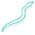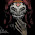## Thursday, November 11, 2010

### Bridge

Today I released a new version of the Bridge script, which brings some new features to the old script. You can read the information for artists on the bridge-page of my website, or visit the BlenderArtists thread. This blogpost however is going to take a deeper look into the code behind the changes. More specifically I'm going to discuss the Cubic Interpolation.

Cubic Interpolation

One of the new features in the script is an interpolation type called 'cubic'. This makes use of natural cubic splines, the same method I used in the LoopTools script.As you can see in the image on the left this results in fluid curves which can be very useful for organic modelling. The problem with natural cubic curves though is that you need more than two input coordinates.

The first two coordinates that are used are pretty straightforward. They are the location coordinates of the vertices that need to be connected to each other. To calculate the spline between them we also need to have two additional input coordinates, one on each side of the vertices. In order to make this a bit more understandable let's take a look at the following example.

The example input is shown in the top image on the right. V1 and V2 are the input vertices. Between them I've drawn the result we wish to get; the spline. In order to get it we need the two additional input points: A1 and A2.

The first step in obtaining A1 and A2 are calculating the tangent vectors of all the edges in the loops. To do this you take the Face Normal vector (blue) and calculate the cross product with the edge vector (red). This gives the Cross vector (green). There are actually two Cross vectors, one facing from the edge to the face, and one facing from the edge to the opposite loop (displayed in the image). We can simple check which one we got by adding the vector to the edge-center and see if we're getting closer to the face center or further away from it. We negate the vector as necessary and then normalize it.

The last step is to calculate the Average vector for each vertex. Simply take the Cross vectors from the edges connected to it and take the average. Adding this Average vector to the location of V1 gives us A1. Note: the size of the Average vector is scaled to be the same as the distance between V1 and V2 and multiplied by the strength factor as given by the artist.

This method works well for loops that are connected to faces, but that isn't always the case. For loops that aren't connected to faces there are two changes. First of all, there is no face normal. An alternative is calculated by taking the cross product of the edge vector and the vector connecting V1 and V2. The second difference is that we calculate the spline per vector, instead of first calculating all Cross vectors. This is because an edge is connected to 2 vertices, and both might be connected to different vertices in the other loop (which is used for calculating the alternative to the face normal). The rest of the procedure is more or less the same.

1.Huh, this is something that i really would like to see in every blender backage as a feature. :o

2.I actually have good hopes this will be included to Blender as one of the default Add-Ons.

3.4.Hi, I'd like to use your code as part of a 3D reconstruction system, are there books/papers I could cite other than this blogpost?

5.Dear Crouch,

Sorry to bother you again, but have you had a chance to look into my post?
Any pointers regarding the algorithm? I will start working heavily on it now,
and I may be able to help improve it or maintain it.

Thanks